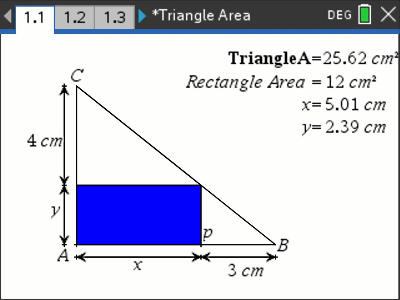Activities

•• AuthorAust Senior

20 Minutes

• Device
•TI-Nspire™ CX
•TI-Nspire™ CX CAS
• Software

TI-Nspire™ CAS
TI-Nspire™

5.0

Triangle AreasActivity Overview

A triangle circumscribes a rectangle. The triangle is not unique, so what is the minimum area of the triangle that circumscribes the triangle? Students use the dynamic representation on TI-Nspire, data capture and more to explore this problem with some elegant solutions.

Objectives

The purpose of this activity is for students to combine some straight forward geometry (similar triangles) to establish a formula for the area of a special triangle so they can use calculus to determine the minimum area.

Vocabulary

• Similar triangles
• Derivative (rational function)
• Minimum